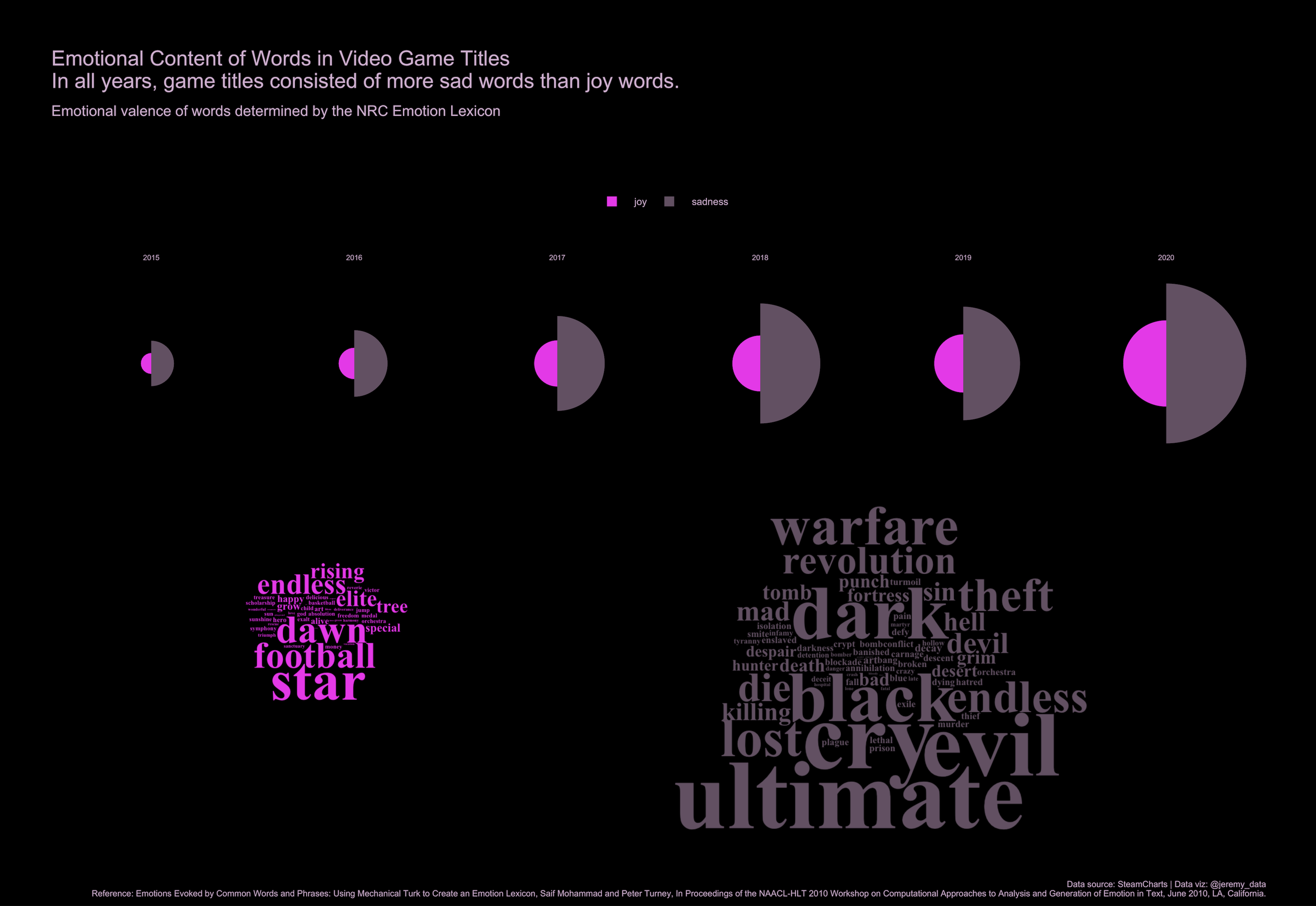# Video Game Title Emotions - a text analysis

Using R to create a data visualization of the emotional content of video game titles

Jeremy Allen https://jeremydata.com
03-20-2021Figure 1: Video Game Title Emotions

## Video Game Title Emotions - a text analysis

This week’s Tidy Tuesday challenge offered video game data for analysis. I analyzed the text in the video game titles. Using the NRC Emotion Lexicon I measured the joy words and sad words in the titles by year.

I used the tidytext package to tokenize each years-worth of game titles and remove stop words and plotted the polar plots with the gpglot2 package. Then I made word clouds using wordcloud2. The clowplot package was used to place screenshots of the wordclouds within the final image.

Code is also on GitHub

``````library(wordcloud2)
library(cowplot)
library(tidyverse)
library(tidytext)

get_sentiments("nrc")

data(stop_words)

nrc_types <- get_sentiments("nrc") %>%
pull(sentiment) %>%
unique()

nrc_joy <- get_sentiments("nrc") %>%
filter(sentiment == "joy")
nrc_sad <- get_sentiments("nrc") %>%
filter(sentiment == "sadness")

stops <- stop_words %>%
bind_rows(tibble(
word = c("edition", "online", "ii", "iii", "iv", "vi", "vii",
"viii", "ix", "definitive", "remastered"),
lexicon = c(rep("jda", 11))
))
``````
``````games <- readr::read_csv('https://raw.githubusercontent.com/rfordatascience/tidytuesday/master/data/2021/2021-03-16/games.csv')
``````
``````# function to get emotion words and output as list of two types
get_emotion_words <- function(x) {

name <- as_tibble(x) %>%
rename(gamename = value) %>%
count(gamename) %>%
mutate(gamename = str_remove_all(gamename, "<U.....>")) %>%
select(-n) %>%
unnest_tokens(
output = word,
input = gamename,
token = "words",
to_lower = TRUE,
strip_punct = TRUE
) %>%
anti_join(stops) %>%
filter(
nchar(word) > 1,              # must be more than one character
str_detect(word, "^[a-z]"),   # must start with a letter
str_detect(word, "[a-z]")     # must not be only numbers
) %>%
count(word, sort = TRUE) %>%
mutate(word = reorder(word, n))

emotion_words <- list(
joy = name %>% inner_join(nrc_joy),
sad = name %>% inner_join(nrc_sad)
)

emotion_words

}

name_emotions <- games %>%
filter(year > 2014 & year < 2021) %>%
group_by(year) %>%
summarise(sum_avg_players = sum(avg),
emotion_words = get_emotion_words(gamename)) %>%
ungroup() %>%
arrange(year)

emotions_scored <- name_emotions %>%
unnest(emotion_words) %>%
mutate(score = n*sum_avg_players) %>%
group_by(year, sentiment) %>%
summarise(valence = sum(score)) %>%
ungroup() %>%
mutate(sentiment = factor(sentiment, levels = c("sadness", "joy"), order = TRUE))
``````
``````joy_title_words <- name_emotions %>%
unnest(emotion_words) %>%
select(word, sentiment, freq = n) %>%
filter(sentiment == "joy") %>%
select(-sentiment) %>%
group_by(word) %>%
summarise(freq = sum(freq))

sad_title_words <- name_emotions %>%
unnest(emotion_words) %>%
select(word, sentiment, freq = n) %>%
filter(sentiment == "sadness") %>%
select(-sentiment) %>%
group_by(word) %>%
summarise(freq = sum(freq))
``````
``````# open these in the viewer pane then screenshot and add screenshot to directory
joy_cloud <- wordcloud2(
data = joy_title_words,
size = .7,
gridSize = 1,
color = "#e600e6",
backgroundColor = "black",
minRotation = 0,
maxRotation = 0,
rotateRatio = 0,
shape = "circle"
)

sad_cloud <- wordcloud2(
data = sad_title_words,
size = 1.1,
gridSize = 1,
color = "#625062",
backgroundColor = "black",
minRotation = 0,
maxRotation = 0,
rotateRatio = 0,
shape = "circle"
)
``````
``````p <- emotions_scored %>%
ggplot(aes(x = sentiment, y = valence, fill = sentiment)) +
geom_bar(stat = "identity", width = 1) +
scale_fill_manual(values = c("#e600e6", "#625062"), breaks = c("joy", "sadness")) +
labs(x = "",
y = "",
title = "Emotional Content of Words in Video Game Titles
In all years, game titles consisted of more sad words than joy words.",
subtitle = "Emotional valence of words determined by the NRC Emotion Lexicon",
caption = "Data source: SteamCharts | Data viz: @jeremy_data
Reference: Emotions Evoked by Common Words and Phrases: Using Mechanical Turk to Create an Emotion Lexicon, Saif Mohammad and Peter Turney, In Proceedings of the NAACL-HLT 2010 Workshop on Computational Approaches to Analysis and Generation of Emotion in Text, June 2010, LA, California.",
fill = "") +
coord_polar() +
facet_wrap(~ year, ncol = 6) +
theme_minimal() +
theme(
text = element_text(color = "#cfafcf"),
strip.text = element_text(size = 12, color = "#cfafcf"),
plot.title = element_text(size = 34, margin = margin(0,0,.25,0, unit = "cm")),
plot.subtitle = element_text(size = 24, margin = margin(.5,0,4,0, unit = "cm")),
plot.caption = element_text(size = 14, margin = margin(24,0,0,0, unit = "cm")),
legend.box.margin = margin(.25,0,2,0, unit = "cm"),
legend.position = "top",
legend.text = element_text(size = 16),
legend.spacing.x = unit(1, 'cm'),
legend.text.align = 0,
panel.grid = element_blank(),
axis.title.x = element_blank(),
axis.title.y = element_blank(),
axis.text.x = element_blank(),
axis.text.y = element_blank(),
plot.margin = margin(3,3,.5,3, unit = "cm"),
plot.background = element_rect(fill = "black"),
)

q <- ggdraw(p) +
draw_image(here::here("joycloud.png"), x = .125, y = .265, hjust = 0, vjust = 0, width = 0.25, height = 0.15) +
draw_image(here::here("sadcloud.png"), x = .48, y = .12, hjust = 0, vjust = 0, width = 0.37, height = 0.37)

ggsave("sad_games.png", plot = q, width = 30, height = 26, units = "in")
``````

### Reuse

Text and figures are licensed under Creative Commons Attribution CC BY 4.0. The figures that have been reused from other sources don't fall under this license and can be recognized by a note in their caption: "Figure from ...".

### Citation

For attribution, please cite this work as

`Allen (2021, March 20). jeremydata: Video Game Title Emotions - a text analysis. Retrieved from https://jeremydata.com/posts/2021-03-20-video-game-title-emotions-a-text-analysis/`

BibTeX citation

```@misc{allen2021video,
author = {Allen, Jeremy},
title = {jeremydata: Video Game Title Emotions - a text analysis},
url = {https://jeremydata.com/posts/2021-03-20-video-game-title-emotions-a-text-analysis/},
year = {2021}
}```